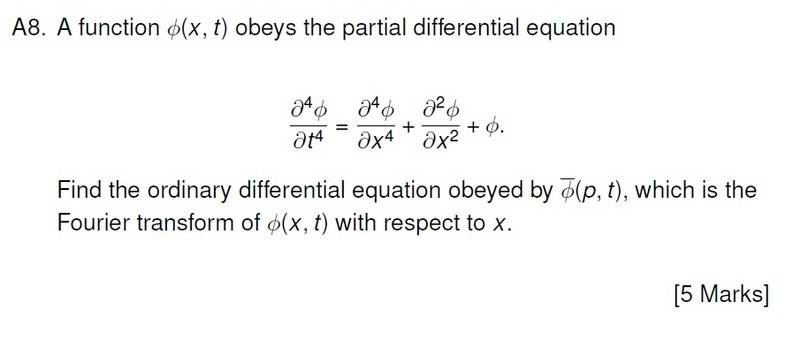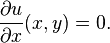# Fourier Transform with PDE

• sa1988

## Homework Statement## The Attempt at a Solution

First write ##\phi(x,t)## as its transform

##\frac{1}{\sqrt{2\pi}}\int_{-\infty}^{\infty} \! e^{ipx} \widetilde{\phi}(p,t) \, \mathrm{d}p##

which I then plug into the PDE in the question to get:

##\frac{1}{\sqrt{2\pi}}\int_{-\infty}^{\infty} \! e^{ipx}\frac{\partial^4\widetilde{\phi}(p,t)}{\partial t^4} \, \mathrm{d}p = \frac{1}{\sqrt{2\pi}}\int_{-\infty}^{\infty} \! e^{ipx}(p^4-p^2) \widetilde{\phi}(p,t) \, \mathrm{d}p##

Which neatens to:

##\frac{1}{\sqrt{2\pi}}\int_{-\infty}^{\infty} \! e^{ipx}\Big[\frac{\partial^4\widetilde{\phi}(p,t)}{\partial t^4}-(p^4-p^2) \widetilde{\phi}(p,t)\Big] \, \mathrm{d}p = 0##

which can only hold if the part in the square bracket obeys

##\frac{\partial^4\widetilde{\phi}(p,t)}{\partial t^4}=(p^4-p^2) \widetilde{\phi}(p,t)##

So this is where I am now. I've found a differential equation obeyed by ##\widetilde{\phi}(p,t)##, however it's a PDE, not an ODE. So I'm either mistaken with my understanding of what the precise definition of an ODE is, or I've gone wrong with my logic in the working out.

To be honest the wording of the question itself seem a touch ambiguous. Is it even possible for a multi-variable function to be part of an ODE?

Any help would be appreciated, thanks.

## Homework Statement## The Attempt at a Solution

First write ##\phi(x,t)## as its transform

##\frac{1}{\sqrt{2\pi}}\int_{-\infty}^{\infty} \! e^{ipx} \widetilde{\phi}(p,t) \, \mathrm{d}p##

which I then plug into the PDE in the question to get:

##\frac{1}{\sqrt{2\pi}}\int_{-\infty}^{\infty} \! e^{ipx}\frac{\partial^4\widetilde{\phi}(p,t)}{\partial t^4} \, \mathrm{d}p = \frac{1}{\sqrt{2\pi}}\int_{-\infty}^{\infty} \! e^{ipx}(p^4-p^2) \widetilde{\phi}(p,t) \, \mathrm{d}p##

Which neatens to:

##\frac{1}{\sqrt{2\pi}}\int_{-\infty}^{\infty} \! e^{ipx}\Big[\frac{\partial^4\widetilde{\phi}(p,t)}{\partial t^4}-(p^4-p^2) \widetilde{\phi}(p,t)\Big] \, \mathrm{d}p = 0##

which can only hold if the part in the square bracket obeys

##\frac{\partial^4\widetilde{\phi}(p,t)}{\partial t^4}=(p^4-p^2) \widetilde{\phi}(p,t)##

So this is where I am now. I've found a differential equation obeyed by ##\widetilde{\phi}(p,t)##, however it's a PDE, not an ODE. So I'm either mistaken with my understanding of what the precise definition of an ODE is, or I've gone wrong with my logic in the working out.

To be honest the wording of the question itself seem a touch ambiguous. Is it even possible for a multi-variable function to be part of an ODE?

Any help would be appreciated, thanks.

Your final equation is an ordinary differential equation, NOT a partial differential equation. You can tell that from the fact that the only derivative involved is taken with respect to ##t##, so you could (and should) write ##d/dt## instead of ##\partial/\partial t##. (You would have had a partial differential equation if you had ##\partial / \partial p## involved as well, but you do not.)

•sa1988
Your final equation is an ordinary differential equation, NOT a partial differential equation. You can tell that from the fact that the only derivative involved is taken with respect to ##t##, so you could (and should) write ##d/dt## instead of ##\partial/\partial t##. (You would have had a partial differential equation if you had ##\partial / \partial p## involved as well, but you do not.)

Aaahh ok, so it only becomes a PDE when another partial differential is actually involved.

I thought it was PDE because it only dealt with one variable on a multi-variable function.

Thanks for clearing that up.

EDIT:

Having said that... the stock definition on wikipedia seems to say that my solution is a PDE..?

In mathematics, a partial differential equation (PDE) is a differential equation that contains unknown multivariable functions and their partial derivatives.

It goes on to say,
A relatively simple PDE isIs this not pretty much the same as my answer above?

Aaahh ok, so it only becomes a PDE when another partial differential is actually involved.

I thought it was PDE because it only dealt with one variable on a multi-variable function.

Thanks for clearing that up.

EDIT:

Having said that... the stock definition on wikipedia seems to say that my solution is a PDE..?

It goes on to say,

Is this not pretty much the same as my answer above?

Yes, but you are getting hung up on things that really do not matter! Whether you write ##\partial u(x,y) /\partial x = 0## or ##du(x,y)/dx = 0## depends on whether or not you regard ##y## as a variable (having the same type of status as ##x##) or as just a parameter that appears in the formula. For example, if I write ##f(x) = a x^2 + bx + c##, I would normally regard ##a,b,c## as some type of input parameters, and ##x## as the only variable involved. So, I would write ##df/dx## for the derivative, because the idea is that ##y = ax^2+ bx + c## is a curve in ##(x,y)##-space, and for fixed ##a,b,c## corresponds to a single fixed curve, about which I might ask several questions about aspects involving different values of ##x## (such its ##x##-intercepts, its maximum or minimum points and the like). For each input curve the parameters ##a,b,c## remain the same throughout the calculations.

•sa1988
Yes, but you are getting hung up on things that really do not matter!

One does not learn without getting hung up on the details every now and again!

Thanks for clearing it up